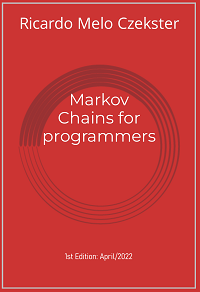# markov

Materials for book: "Markov Chains for programmers"

View the Project on GitHub czekster/markov

# Book: “Markov Chains for programmers”This book was published by Self Publishers Worldwide (another publication by the lazy panda collection), and authored by Ricardo M. Czekster.

The first edition was made public in April/2022.

“Markov Chains for programmers” is devoted to programmers at any level wanting to understand more about the underpinnings of Markov Chains (MC) and basic solution methods.

You will find here supporting materials for the book such as C programming code and solutions, MATLAB scripts, PRISM models (CTMC/DTMC) for the examples provided in the book, which you may also download here.

This book is a companion to this paper about Performance Evaluation, published as a pre-print in ResearchGate.

The book is under Creative Commons (CC BY 4.0) and the code is under GPLv3.

Feel free to use it and attribute the original source.

## Folders

The structure of the repository is as follows:

• `challenges`: proposed challenges throughout the book;
• `matlab`: MATLAB® scripts for some solutions;
• `models`: a list of DTMC and CTMC models (in the format that we explored in the book, with code);
• `prism`: PRISM models (in CTMC and DTMC);
• `spreadsheets`: MS-Excel spreadsheets reproducing the experiments (CTMC/DTMC solution, simulation);

## Challenges

The book proposes a number of challenges throughout its chapters. The code for all solutions is available for download in this repository.

One remark: to avoid repetitions in the code, there is a folder called `project00` that has a `Makefile` and all the challenges (you may want to check the file `markov.c` to access auxiliary functions). In this project, each challenge has a `main()` function, so they work independently.

The `project01` folder contains a full project with one `main()` function and several built-in command-line options. Consult “Challenge 07” for more information about this project.

Please, let me know if you find something odd or something that needs changing. Thank you in advance.

## Models

The book offers many models for evaluating your projects in a textual matrix format (.txt), prism (.sm or .pm), and MATLAB (.m).

### CTMC/DTMC models to run internally

The following models are available (they were named SN where N is the number of states):

• `models/S2-lighting-ctmc.txt`: The Lighting model, using rates;
• `models/S2-lighting-dtmc.txt`: The Lighting model, using probabilities;
• `models/S2-pushkin-dtmc.txt`: The pushkin model developed by A. A. Markov (according to Grinstead and Snell’s book Introduction to Probability);
• `models/S3-land-of-oz-dtmc.txt`: The land of oz model;
• `models/S3-weather-belfast-dtmc.txt`: The Belfast weather model (by Stewart);
• `models/S4-aging-rejuvenation-ctmc`: Software aging and rejuvenation model, CTMC;
• `models/S4-aging-rejuvenation-dtmc`: Same (aging & rej), DTMC version;
• `models/S4-generic-ctmc.txt`: The Generic model;
• `models/S4-lilypad-ctmc.txt`: The Lily Pad model (frog in the pond);
• `models/S4-maze-model-dtmc.txt`: The Mouse Maze model;
• `models/S5-ehrenfest-dtmc.txt`: The Ehrenfest model;
• `models/S6-birth-and-death-ctmc.txt`: A six state Birth and Death model;
• `models/S9-maze-model-dtmc.txt`: A larger Mouse Maze model, with 9 states;
• `models/S26-shakespeare-ctmc.txt`: A brief analysis of one of Shakespeare’s Sonnets with MC;

### PRISM models

• `prism/belfast-dtmc.pm` for the Belfast weather model;
• `prism/generic-ctmc.sm` for the Generic model;
• `prism/lily-ctmc.sm` and `lily.dtmc.pm` for the Lily pad model;
• `prism/aging.sm` and accompanying `prism/aging.props` model and property file, respectively;

### MATLAB models

• `matlab/generic_model_dtmc.m`;
• `matlab/matlabsolution_dtmc.m`;

## Challenges

You may try to run the challenges code for these models (beware that the type should match, either CTMC or DTMC). Click here for more information on models

## Numerical methods, models and tools

The methods, models and tools explored in the book are Power Matrix, Vector-Matrix Multiplication, PRISM models, some MATLAB® scripting, forward simulation, and some MS-Excel spreadsheets (with basic models).

## How to cite the workRicardo M. Czekster rczekster at gmail